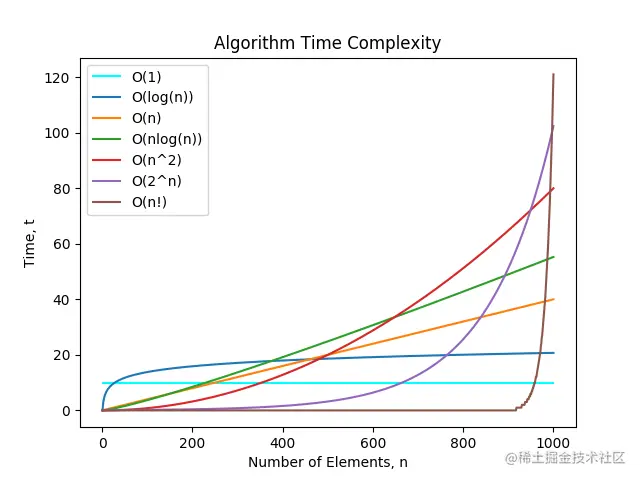### # 时间复杂度

• `O(1)`: Constant Complexity: Constant 常数复杂度
• `O(log n)`: Logarithmic Complexity: 对数复杂度
• `O(n)`: Linear Complexity: 线性时间复杂度
• `O(n^2)`: N square Complexity 平⽅方
• `O(n^3)`: N square Complexity ⽴立⽅方
• `O(2^n)`: Exponential Growth 指数
• `O(n!)`: Factorial 阶乘